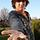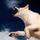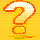## General Question# How can I prove that something converges using the limit comparison test when I can't seem to find something to which it compares?

Asked by HeroicZach (195) October 28th, 2010

I’m working with (ln(n))^3 / n^3. No matter what I attempt to compare this function to, it always results in a failure of the test – either an / bn converges while the summation of bn diverges or vice versa. Is there something I’m missing here?

Observing members: 0Composing members: 0Um… I did this sort of maths too long ago… But did you consider the fact that both the denominator and numerator share the same ^3 thing? So you can take it outside?

In other words, if you’re finding limit of [(ln n)/n]^3, as n approaches infinity. And doesn’t the limit of (ln n)/n approach a definite value as n tends to infinity?

Hopefully this will point you in the right direction. Unless I’m completely wrong about it, in which case I do apologise.

genkan (784)“Great Answer” (0) Flag as…I am assuming you are talking about testing the series sum (ln(n))^3 / n^3 for convergence.

Compare this series with 1/n^2. Let me know if you can’t figure it out.

BonusQuestion (1482)“Great Answer” (2) Flag as…or